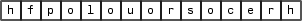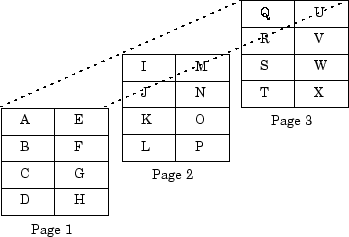Documentation

## Data Handling

### MATLAB Array

The MATLAB® Runtime works with a single object type: the MATLAB array. All MATLAB variables (including scalars, vectors, matrices, character arrays, cell arrays, structures, and objects) are stored as MATLAB arrays. In the MATLAB Production Server™ C/C++ client API, the MATLAB array is declared to be of type `mpsArray`. The `mpsArray` structure contains the following information about the array:

• Type

• Dimensions

• Data associated with the array

• If numeric, whether the variable is real or complex

• If sparse, its indices and nonzero maximum elements

• If a structure or object, the number of fields and field names

To access the `mpsArray` structure, use the `mpsArray` API functions. These functions enable you to create, read, and query information about the MATLAB data used by the client.

### Note

The `mpsArray` API mirrors the `mxArray` API used by MATLAB Compiler SDK™ and MATLAB external interfaces.

### Data Storage

MATLAB stores data in a column-major (columnwise) numbering scheme. MATLAB internally stores data elements from the first column first, then data elements from the second column second, and so on, through the last column.

For example, given the matrix:

```a=['house'; 'floor'; 'porch'] a = house floor porch```

its dimensions are:

```size(a) ans = 3 5```

and its data is stored as:If a matrix is N-dimensional, MATLAB represents the data in N-major order. For example, consider a three-dimensional array having dimensions 4-by-2-by-3. Although you can visualize the data as:MATLAB internally represents the data for this three-dimensional array in the following order:

 A B C D E F G H I J K L M N O P Q R S T U V W X 0 1 2 3 4 5 6 7 8 9 10 11 12 13 14 15 16 17 18 19 20 21 22 23

The `mpsCalcSingleSubscript()` function creates the offset from the first element of an array to the desired element, using N-dimensional subscripting.

### Note

MATLAB indexing starts at 1 where C indexing starts at 0.

### MATLAB Types

#### Complex Double-Precision Matrices

Complex double-precision, non-sparse matrices are of type double and have dimensions m-by-n, where `m` is the number of rows and `n` is the number of columns. The data is stored as two vectors of double-precision numbers—one contains the real data and one contains the imaginary data. The pointers to this data are referred to as `pr` (pointer to real data) and `pi` (pointer to imaginary data), respectively. A non-complex matrix is one whose `pi` is `NULL`.

#### Numeric Matrices

Numeric matrices are single-precision floating-point integers that can be 8-, 16-, 32, and 64-bit, both signed and unsigned. The data is stored in two vectors in the same manner as double-precision matrices.

#### Logical Matrices

The `logical` data type represents a logical `true` or `false` state using the numbers `1` and `0`, respectively. Certain MATLAB functions and operators return logical `1` or logical `0` to indicate whether a certain condition was found to be true or not. For example, the statement ```(5 * 10) > 40``` returns a logical `1` value.

#### MATLAB Character Arrays

MATLAB character arrays are of type `char` and are stored in a similar manner as unsigned 16-bit integers, except there is no imaginary data component. Unlike C, MATLAB character arrays are not null terminated.

#### Cell Arrays

Cell arrays are a collection of MATLAB arrays where each `mpsArray` is referred to as a cell, enabling MATLAB arrays of different types to be stored together. Cell arrays are stored in a similar manner to numeric matrices, except the data portion contains a single vector of pointers to `mpsArray`s. Members of this vector are called cells. Each cell can be of any supported data type, even another cell array.

#### Structures

Structures are MATLAB arrays with elements accessed by textual field designators.

Following is an example of how structures are created in MATLAB:

```S.name = 'Ed Plum'; S.score = 83; S.grade = 'B+'```

creates a scalar structure with three fields:

``` S = name: 'Ed Plum' score: 83 grade: 'B+' ```

A 1-by-1 structure is stored in the same manner as a 1-by-`n` cell array where `n` is the number of fields in the structure. Members of the data vector are called fields. Each field is associated with a name stored in the `mpsArray`.

#### Multidimensional Arrays

A multidimensional array is a vector of integers where each element is the size of the corresponding dimension. The storage of the data is the same as matrices. MATLAB arrays of any type can be multidimensional.

#### Empty Arrays

MATLAB arrays of any type can be empty. An empty `mpsArray` is one with at least one dimension equal to zero. For example, a double-precision `mpsArray` of type `double`, where `m` and `n` equal `0` and `pr` is `NULL`, is an empty array.

#### Sparse Matrices

Sparse matrices have a different storage convention from that of full matrices in MATLAB. The parameters `pr` and `pi` are still arrays of double-precision numbers, but these arrays contain only nonzero data elements. There are three additional parameters:

• `nzmax` is an integer that contains the length of `ir`, `pr`, and, if it exists, `pi`. It is the maximum number of nonzero elements in the sparse matrix.

• `ir` points to an integer array of length `nzmax` containing the row indices of the corresponding elements in `pr` and `pi`.

• `jc` points to an integer array of length `n+1`, where n is the number of columns in the sparse matrix. The `jc` array contains column index information. If the `j`th column of the sparse matrix has any nonzero elements,` jc[j]` is the index in `ir` and `pr` (and `pi` if it exists) of the first nonzero element in the `j`th column, and `jc[j+1] - 1` is the index of the last nonzero element in that column. For the `j`th column of the sparse matrix, `jc[j]` is the total number of nonzero elements in all preceding columns. The last element of the `jc` array, `jc[n]`, is equal to `nnz`, the number of nonzero elements in the entire sparse matrix. If `nnz` is less than `nzmax`, more nonzero entries can be inserted into the array without allocating more storage.

### Using Data Types

You can write MATLAB Production Server client applications in C/C++ that accept any class or data type supported by MATLAB (see MATLAB Types).

### Caution

The MATLAB Runtime does not check the validity of MATLAB data structures created in C/C++. Using invalid syntax to create a MATLAB data structure can result in unexpected behavior.

#### Declaring Data Structures

To handle MATLAB arrays, use type `mpsArray`. The following statement declares an `mpsArray` named `myData`:

```mpsArray *myData; ```

To define the values of `myData`, use one of the `mpsCreate*` functions. Some useful array creation routines are `mpsCreateNumericArray()`, `mpsCreateCellArray()`, and `mpsCreateCharArray()`. For example, the following statement allocates an `m`-by-1 floating-point `mpsArray` initialized to `0`:

`myData = mpsCreateDoubleMatrix(m, 1, mpsREAL);`

C/C++ programmers should note that data in a MATLAB array is in column-major order. (For an illustration, see Data Storage.) Use the `mpsGet*` array access routines to read data from an `mpsArray`.

#### Manipulating Data

The `mpsGet*` array access routines get references to the data in an `mpsArray`. Use these routines to modify data in your client application. Each function provides access to specific information in the `mpsArray`. Some useful functions are `mpsGetData()`, `mpsGetPr()`, `mpsGetM()`, and `mpsGetString()`. The following statements read the input character array`prhs` into a C-style string `buf`:

```char *buf; int buflen; int status; buflen = mpsGetN(prhs)*sizeof(mpsChar)+1; buf = malloc(buflen); status = mpsGetString(prhs, buf, buflen);```
##### Support평가판 신청